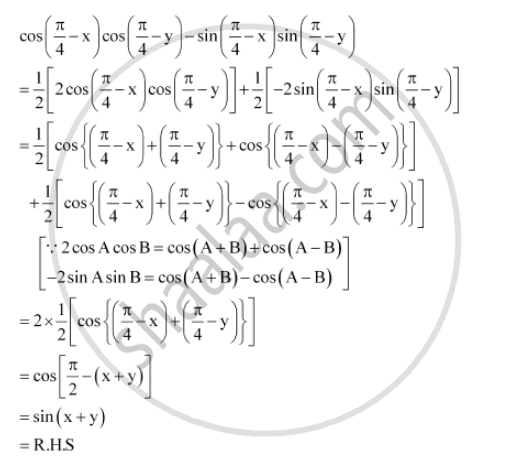CBSE (Arts) Class 11CBSE
Share

# Prove That: Cos (Pi/4 Xx X) Cos (Pi/4 - Y) - Sin (Pi/4 - X)Sin (Pi/4 - Y) = Sin (X + Y) - CBSE (Arts) Class 11 - Mathematics

ConceptTrigonometric Functions of Sum and Difference of Two Angles

#### Question

Prove that: cos (pi/4 xx x) cos (pi/4 - y) - sin (pi/4 -  x)sin (pi/4  - y) =  sin (x + y)

#### SolutionIs there an error in this question or solution?

#### APPEARS IN

NCERT Solution for Mathematics Textbook for Class 11 (2018 to Current)
Chapter 3: Trigonometric Functions
Q: 6 | Page no. 73

#### Video TutorialsVIEW ALL 

Solution Prove That: Cos (Pi/4 Xx X) Cos (Pi/4 - Y) - Sin (Pi/4 - X)Sin (Pi/4 - Y) = Sin (X + Y) Concept: Trigonometric Functions of Sum and Difference of Two Angles.
S